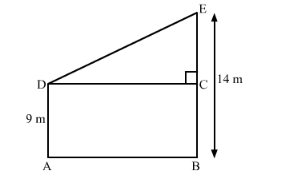# Two poles of height 9 m and 14 m stand on a plane ground.

Question:

Two poles of height 9 m and 14 m stand on a plane ground. If the distance between their feet is 12 m, find the distance between their tops.

Solution:

Let us draw the diagram from the given information.As we are given that distance between their feet is 12 m

$\therefore D C=12$.

Now we get a right angled triangle DCE.

Let us applying the Pythagoras theorem we get,

$D E^{2}=D C^{2}+E C^{2}$

Substituting the values we get,

$D E^{2}=12^{2}+5^{2}$

$D E^{2}=144+25$

$\therefore D E^{2}=169$

Let us take the square root we get,

$\therefore D E=13$

Therefore, distance between their top is 13m.